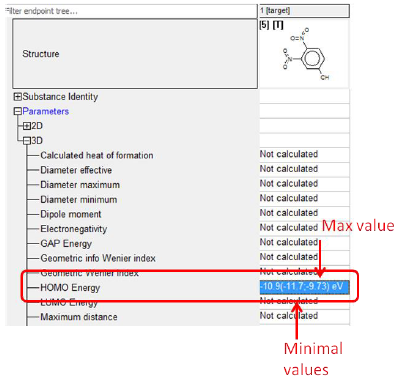The columns with min and max values include minimal and maximal values related to the conformeric forms of the target molecule. In case the molecule is flexible (e.g. the chemical provided on Figure 1), the values are different otherwise the values are same.Figure 1. Calculated parameters for the flexible chemical

In case the target chemical is presented as a set (tautomeric set, metabolism set, mixture) TB provides min and max values calculated for the tautomeric set (Figure 2).Figure 2. Calculation of HOMO Energy for tautomeric set

The values outside the brackets depend on the used calculated options. By default as a representative value is taken weighted mean value (Figure 3).Figure 3

This is due to the fact that the components of the set include quantities. In case there are no quantities available, it is assumed that the quantities are equal to 1.